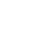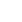# Fractions, Decimals, Percents, & Graphs

Brittany Zae
Teacher

This lesson is designed to explore fractions, decimals, percent’s, and graphs with TRIX cereal.

##Length of Time: About 45 Minutes

### Common Core Alignment

CCSS:MATH.CONTENT.5.NF.A.2 - Solve word problems involving addition and subtraction of fractions referring to the same whole, including cases of unlike denominators, e.g., by using visual fraction models or equations to represent the problem. Use benchmark fractions and number sense of fractions to estimate mentally and assess the reasonableness of answers. For example, recognize an incorrect result 2/5 + 1/2 = 3/7, by observing that 3/7 < 1/2.

## Objectives & Outcomes

Students will be able to calculate fractions from a set of objects, convert fractions to decimals and percent’s, develop a graph based on their individual information and compare/contrast it with others in their cooperative groups

## Materials Needed

• bingo chips
• large paper for chart making, 3
• 9″ x 12″ drawing paper
• box of TRIX
• small handful of TRIX in a ziplock for each student
• compasses
• pencils
• colored pencils
• markers
• crayons

## Procedure

### Opening to Lesson

Review prior information- Students should be familiar with what a fraction, a decimal, and a percent are and why and how we use them. They have had prior experience composing fractions from sets of data.

### Body of Lesson

#### Direct Teaching

Teacher uses magnetic bingo chips (5 blue, 3 red, 4 purple) to illustrate how to find a fraction. Example: 3/12 are red.

Then, using a chart, the teacher leads the students through the process of finding the equivalent decimal and percent for each color. (See #3 & #4)

To find the equivalent decimal the student is shown (on the overhead calculator) how to divide the numerator by the denominator. If a more advanced calculator is available, the teacher could show how to use the fraction to decimal function key.

#### Guided Practice 1

Teacher states that it is time for the students to show their knowledge of this process. Teacher states that the TRIX are needed for the whole activity. So, students are not to enjoy them until they are told to do so. Pass out TRIX supplies and paper.

Students will sort their TRIX by color, tally them, and total them on the handout.

Students will then write the number of orange TRIX (numerator) over the number of total TRIX (denominator) to produce the fraction of orange TRIX. They will then calculate the decimal and percent of each (see #3 and #4 above).

The students will repeat the procedure with their red, blue, green, purple and yellow TRIX.

While the students are working on their individual sets of TRIX’s, they may ask a group member for help if needed, and the teacher is circulating and checking for understanding.

#### Guided Practice 2

When students have completed their tables, the teacher once again calls their attention to the earlier presentation with the bingo chips. The teacher shows them that the decimals found with all the colors should add up to near 1. Students add their decimals and write down the sum. Have students brainstorm why they might not have gotten 1 exactly. (ex: Did they round any of their decimals?)

Again, call the class’ attention to the percent line of the bingo chip table. Add the percents up on the overhead calculator. The sum should be 100%. Have students calculate their total percentages. What did they get? Discuss why/why not they might have gotten 98% or 101%. Discuss how it is related to their answer with the decimals.

#### Independent Practice

Now students are ready to proceed with the graph. Teacher asks that they make a circle, using a compass for each color of their Trixs on a sheet of drawing paper

Now that their circles are complete, the students will use rulers to draw 3 straight lines in the middle of their circles. For example, one might draw a line in the upper third. Then, draw a straight line in the middle of the circle. And then the lower third of the circle. Do this for every the circle representing all of the colors.

The student has now drawn a graph. Have each student label the sections with the color and the fraction, decimal and percentage.

Students may now add a title and color in their graphs. They may also eat their TRIX at this stage "to give them energy."

### Closing

When completed, have students in cooperative groups discuss their graphs and come up with a list of ten statements comparing their graphs. (Ex: Tony has over 50% red TRIX and Sam only has 10% red). They write these on a piece of chart paper and then share with the class in a short presentation.

Hang the charts and graphs for all to see.

## Assessment & Evaluation

Through guided questions, close monitoring and informal observation; the teacher will be able to assess student’s ability and understanding of the subject being introduced. Through individually assessing during independent practice and reviewing during guided practice the teacher will be able to evaluate students.

## Modification & Differentiation

Students may work in groups or individually in order to complete their assignments. In order to accommodate for all students, allow extra time to review for lower students and extra worksheets to occupy the time of advanced students.

## Related Lesson Plans

##### Exchanging Time

The lesson is used for students to practice basic time measurement, and understanding the basic units of time.

##### Silent Geometry

The students will practice using geometry formulas measuring items in the classroom to find area and volume, radius and circumference, and identify the types of angles.

##### Graph Smile

Pairs of students will survey other students in the school and then graph the results, as well as come to conclusions based on the information.

##### Comparing Multiplication Facts (Hey Tocayo!)

Students will be assigned a number that has various factors and they will find partners with different factors that have the same product.

##